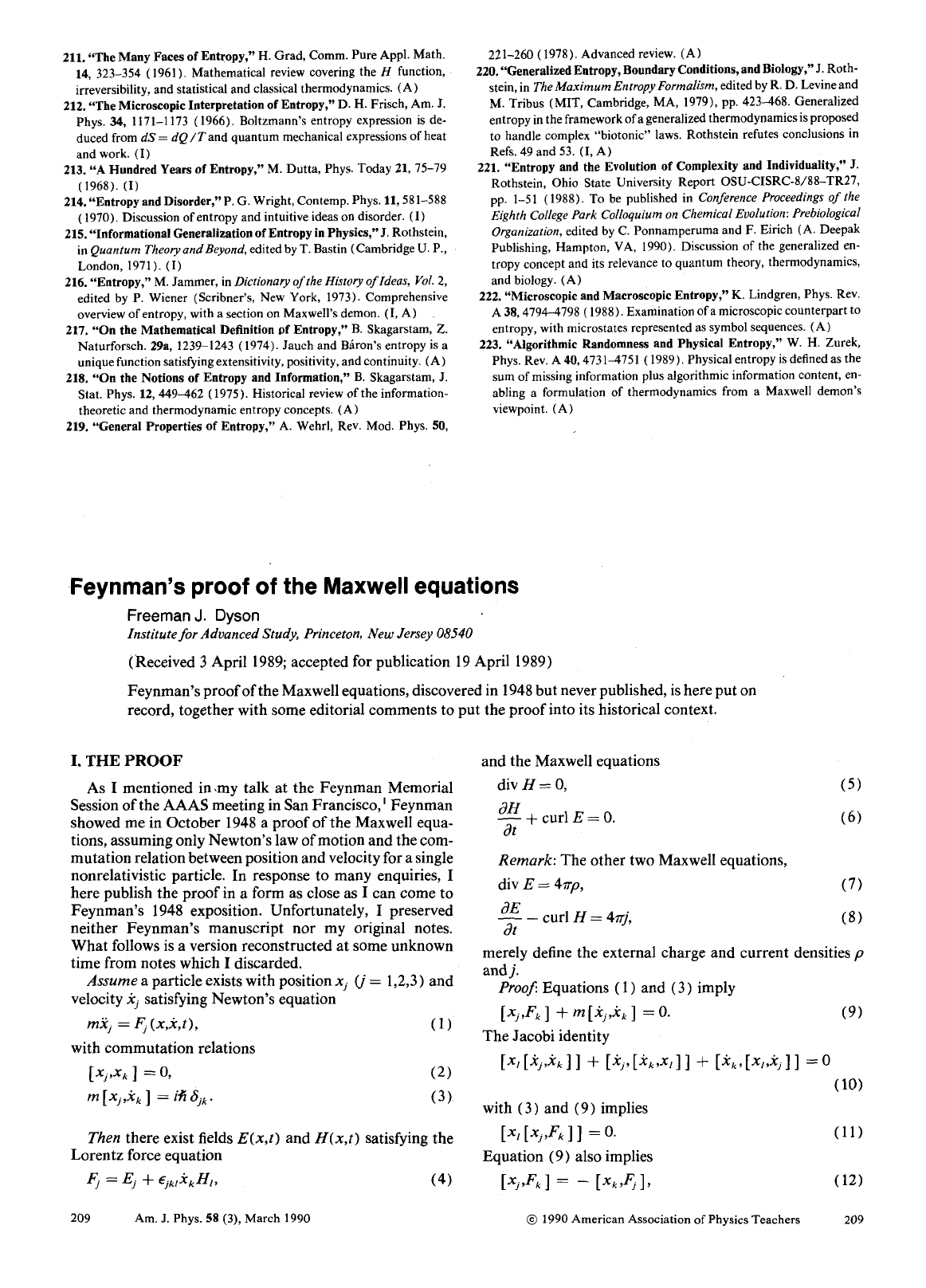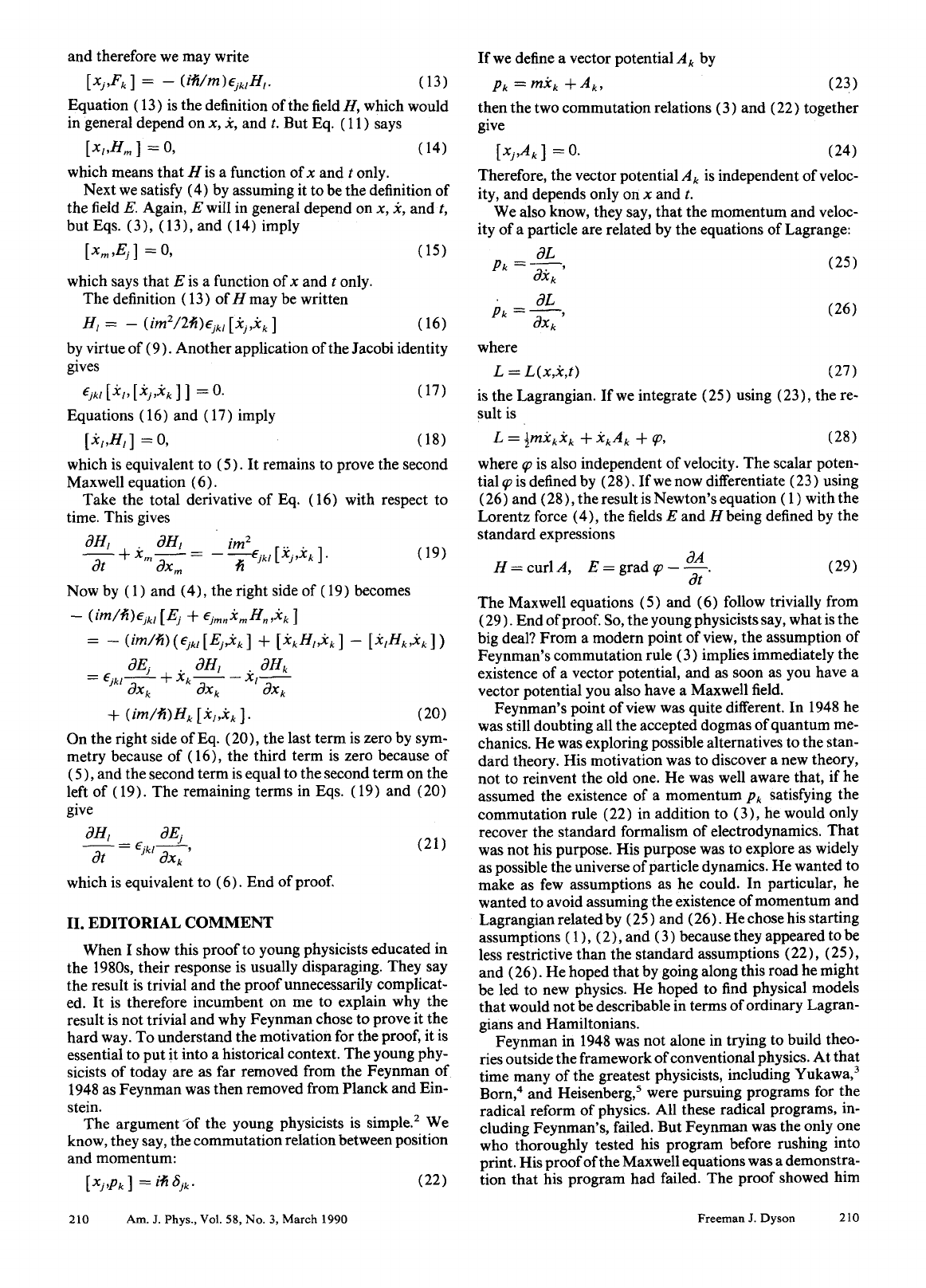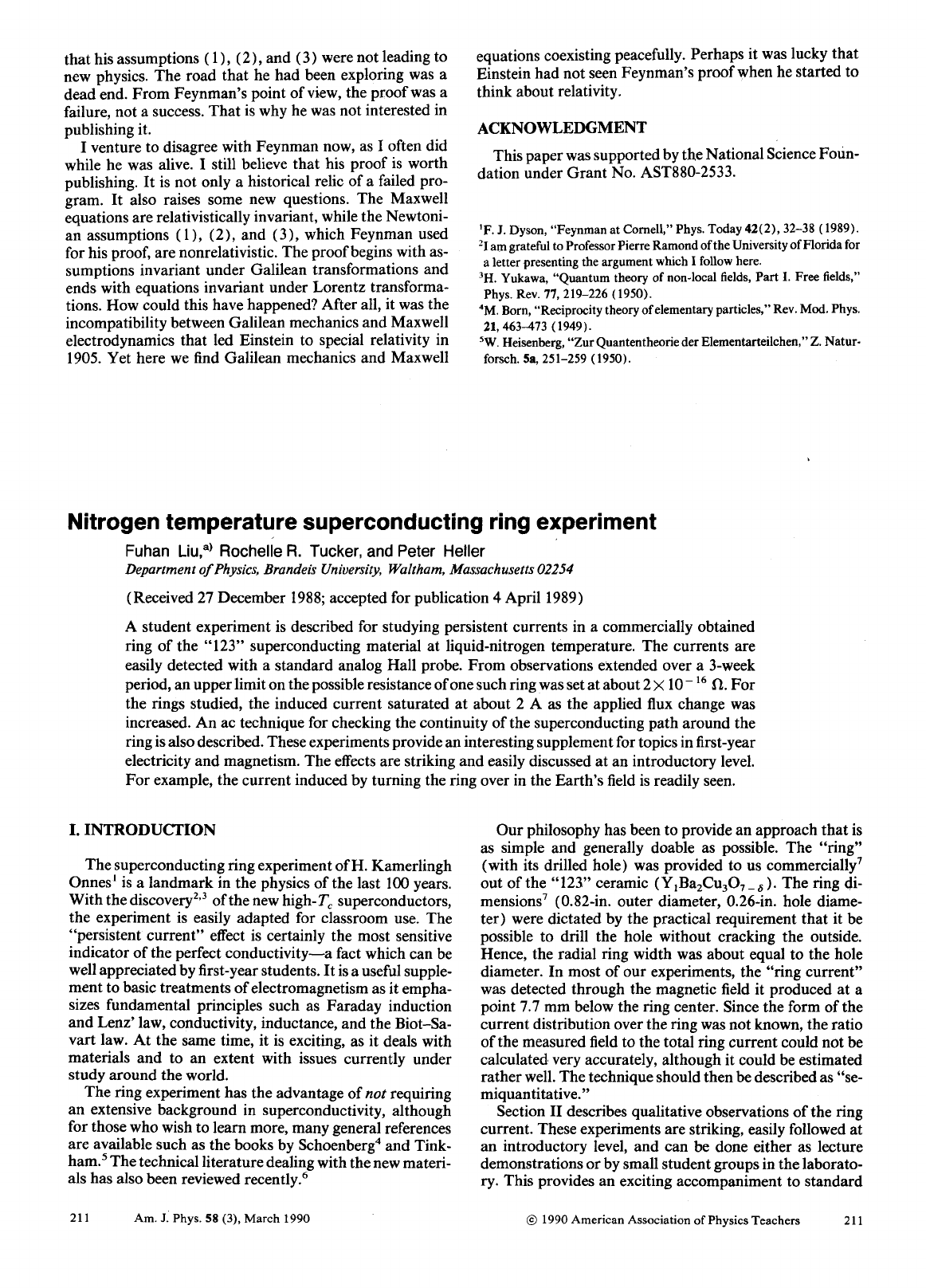Freeman Dyson is an English-born American theoretical physicist and...
There's also an article about Feynman's derivation of the Schröding...
Deriving identity (3) in $t$ $$m\frac{d[x_j,\dot x_{k}]}{dt}=m\f...$$ [x_l,[\dot x_j,\dot x_k]]+[\dot x_l,[\dot x_j,x_k]]+[\dot x_l,[...
$$-[x_k,F_j]=m[\dot x_k, \dot x_j]=-m[\dot x_j, \dot x_k]=[x_j,F_... To prove that [x_j,F_k]=-\frac{i \hbar}{m}\epsilon_{jkl} H_l we f...$$ [x_j,F_k]= -\frac{i\hbar}{m}\epsilon_{jkl}H_l \equiv\\ -m[\dot...
This expression means that $H_l$ is not a function of $x_l$, otherw...
 -\frac{im}{\hbar}\epsilon_{jkl}[E_j+\epsilon_{jmn}\dot x_m H_n ...
Since the space coordinate operators $x_1,x_2,x_3$ commute with eac...
For more on the invariance paradox check the comments below on the ...